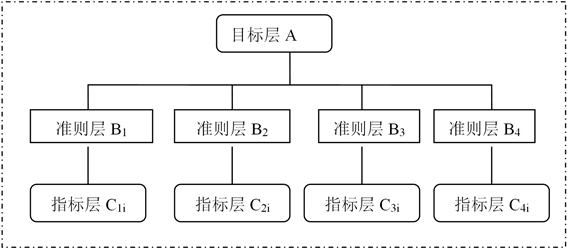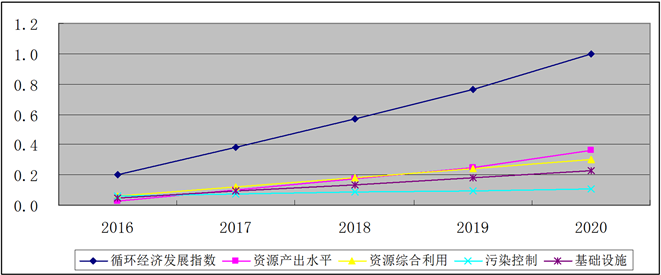﻿ 徐州市循环经济评价指标体系实证研究

# 徐州市循环经济评价指标体系实证研究An Empirical Study on the Evaluation Index System of Circular Economy in Xuzhou

Abstract: Based on the rules of 3R-reducing, reusing and recycling, an evaluation index system of circular economy was proposed by Xuzhou municipal government in its “13th Five-Year” circular economy development plan. This system effectively combines actual needs and then is designed to cover 1 objective layer, 4 principle layers, and 23 index layers. This article made full use of linear weighted synthesis and developed the index of circular economy in the13th Five-Year after the weights of rule layer and index layer were determined by using AHP and entropy method. Furthermore, an implementation path is explored regarding development of circular economy in Xuzhou city.

1. 引言

2015年国家统计局研究建立了循环经济综合评价指标体系，并据此对我国循环经济发展状况进行了测算  ，结果表明：“十二五”期间我国循环经济发展成效明显。2016年徐州市正式获批成为国家循环经济示范市建设城市  ，为此，徐州市政府专门出台了《加快推进国家循环经济示范城市建设实施意见》，全面开启徐州市国家循环经济示范城市建设工作，循环经济的时代已经到来。2016年10月17日，《徐州市“十三五”循环经济发展规划(2016~2020年)》正式印发实施。发展循环经济是徐州市加快转变经济发展方式，建设资源节约型、环境友好型社会，实现可持续发展的必然选择。

20世纪80年代后期德国出现了循环经济的研究和评价，90年代出现大规模实践，以1996年实施的《循环经济与废物管理法》为标志；托宾等人(1972)提出净经济福利指标体系；Daly (1994)提出的“可持续经济福利指数”；Wackernage  (1996)等提出的“生态足迹”概念，即“适当的承载力”。国内学者也从不同角度建立了循环经济指标体系，其中较为典型的有：牛桂敏  (2005)提出了一个循环经济评价体系，该体系由3个层次、6大子模块及36个不同指标构成；钟太洋、黄贤金  等(2006)建立包括资源利用效率、资源减量化利用趋势、污染减量排放、资源再循环与再利用、产业循环结构、能源结构与安全和循环经济发展能力等7个方面的评价体系；计晓东  等(2011)提出循环度概念，以衡量循环经济发展水平。王茂祯  等(2012)提出循环经济创新评价指标体系等。董康银  等(2015)构建循环经济综合指标体系，采用DHGF法对循环经济指标给出有效评价。

2. 徐州市循环经济评价指标体系

2.1. 指标体系设计

“十二五”期间，徐州市循环经济发展成效显著：资源综合利用水平不断提升，循环经济示范试点有序推进，资源节约型社会建设稳步推进，园区循环化改造成效显著，循环经济体制机制不断完善，循环经济意识不断增强。

《徐州市“十三五”循环经济发展规划(2016~2020年)》明确了“十三五”期间徐州市循环经济发展目标。在目标体系上，按照实际和需要相结合的原则，提出了4大类23个发展指标，如表1，其中核心指标资源产出率提高到4000元/吨，比2015年提高18.7%；单位GDP能耗完成国家和省下达指标；单位GDP用水量累计下降15%；单位GDP建设用地占用规模511亩/亿元。Table 1. Xuzhou City’s “13th Five-Year Plan” circular economy development planning index system

2.2. 评价方法   确定

2.2.1. 层次分析法

1) 构造层次分析结构Figure 1. Hierarchical analysis structure of the indicator system

2) 构造判断矩阵

1) ${C}_{ij}>0$

2) ${C}_{ij}=\frac{1}{{C}_{ji}}\left(i\ne j\right)$

3) ${C}_{ii}=1\left(i,j=1,2,\cdots ,n\right)$

Table 3. The scale of the judgment matrix and its meaning

3) 判断矩阵的一致性检验

4) 层次单排序

5) 层次总排序

2.2.2. 熵权算法

$R={\left[\begin{array}{cccc}{r}_{11}& {r}_{12}& \cdots & {r}_{1n}\\ {r}_{21}& {r}_{11}& \cdots & {r}_{2n}\\ ⋮& ⋮& \ddots & ⋮\\ {r}_{m1}& {r}_{m2}& \cdots & {r}_{mn}\end{array}\right]}_{m×n}$ (1)

1) 计算第j个指标下第i个项目的指标值的比重： ${p}_{ij}$

${p}_{ij}={r}_{ij}/\underset{i=1}{\overset{m}{\sum }}{r}_{ij}$ (2)

2) 计算第j个指标的熵值： ${e}_{j}$

${e}_{j}=-k\underset{i=1}{\overset{m}{\sum }}{p}_{ij}\cdot \mathrm{ln}{p}_{ij}$ (3)

3) 计算第j个指标的熵权： ${w}_{j}$

${w}_{j}=\left(1-{e}_{j}\right)/\underset{j=1}{\overset{n}{\sum }}\left(1-{e}_{j}\right)$ (4)

2.2.3. 功效系数法

${{x}^{\prime }}_{ij}=\frac{{x}_{ij}-\mathrm{min}{x}_{ij}}{\mathrm{max}{x}_{ij}-\mathrm{min}{x}_{ij}}\left(0\le {{x}^{\prime }}_{ij}\le 1\right)$ (5)

${{x}^{\prime }}_{ij}=\frac{\mathrm{max}{x}_{ij}-{x}_{ij}}{\mathrm{max}{x}_{ij}-\mathrm{min}{x}_{ij}}\left(0\le {{x}^{\prime }}_{ij}\le 1\right)$ (6)

2.2.4. 线性加权综合法

$y=\underset{j=1}{\overset{m}{\sum }}{w}_{j}\cdot {x}_{j}$ (7)

3. 徐州市循环经济发展水平实证研究

3.1. 数据来源

《徐州市“十三五”循环经济发展规划(2016~2020年)》提出了4大类23个发展指标。目标层为循环经济发展综合评价A，准则层包括资源产出水平B1、资源综合利用B2、污染控制B3、基础设施B4这四个方面。指标层一共有23个指标组成，见表4

Table 4. Indicator layer data (2016-2020)

3.2. 权重确定

1) 准则层权重的确定——AHP

2) 指标层权重的确定——熵权法Table 6. Target layer and indicator layer weights

3.3. 循环经济发展指数计算

3.3.1. 数据的标准化处理Table 7. The satisfaction value and the lowest value of each evaluation index

Table 8. Dimensionless data

3.3.2. 循环经济发展指数的计算Table 9. Xuzhou circular economy development index and criteria layer index (2016-2020)

3.4. 实证小结Figure 2. Xuzhou Circular Economy Development Index and Criteria Layer Index (2016~2020)

4. 徐州市发展循环经济的实施路径

4.1. 建立“四位一体”的循环经济体系

4.2. 推进循环经济体制机制创新

4.3. 优化财政金融政策

2015年国家社科基金项目(15BGL144)，2016年徐州软科学项目(KC16RH132)，徐州工程学院2017年大学生创新创业训练计划项目：“徐州市循环经济发展水平综合评价研究”(XCX2017211)。

 循环经济发展评价指标体系(2017年版) [S].

 徐州市“十三五”循环经济发展规划(2016-2020) [Z].

 Wackernagel, R.W. (1996) Our Eological Foot Print: Reducing Human Pacteaeth. New Society Publisher, Gabriela Island BC.

 牛桂敏. 循环经济评价体系的构建[J]. 城市环境与城市生态, 2005(4): 4-7.

 钟太洋, 黄贤金, 等. 区域循环经济发展评价: 方法, 指标体系与实证研究——以江苏省为例[J]. 资源科学, 2006, 28(2): 154-163.

 计晓东, 杨玉凤. 循环经济的循环度及其相关问题研究[J]. 资源科学, 2011, 33(6): 1126-1130.

 王茂祯, 冯之浚. 循环经济创新评价指标体系研究[J]. 中国人口•资源与环境, 2012, 22(4): 163-167.

 董康银, 孙仁金, 李慧. 炼油企业循环经济综合评价方法研究[J]. 生态经济, 2015, 12(3): 74-79.

 张炳江. 层次分析法及其应用案例[M]. 北京: 电子工业出版社, 2014.

 杜栋, 庞庆华, 吴炎. 现代综合评价方法与案例精选[M]. 北京: 清华大学出版社, 2015.

Top